# How to solve an integral II

TL;DR Summary
How to solve the following integral (in Maple notation): Int(y**k*exp(-u*exp(-y)/a-u*exp(y)/a),y=-infinity..infinity)?
How to solve the following integral (in Maple notation):
Code:
Int(y**k*exp(-u*exp(-y)/a-u*exp(y)/a),y=-infinity..infinity)

with 0<a, 0<u, 0<a, 0<u?

Last edited by a moderator:

Use LaTex!

I am looking for a solution of the following integral

$$\int_{-\infty }^{\infty }\!{y}^{k}{{\rm e}^{-{\frac {u_{{0}} \, {{\rm e}^{-y}}}{a_{{0}}}}-{\frac {u_{{1}} \, {{\rm e}^{y}}}{a_{{1}}}}}}\,{\rm d}y$$
with ## 0<a_{0}##, ## 0<a_{1}##, ## 0<c_{0}##, ## 0<c_{1}## and ##k = 1, 2, 3, ..., ##.

Homework Helper

I find it hard to believe an analytical answer exists.

Doodled with ##\ u_0=a_0 \ \ \& \ \ u_1 = a_1\ ## so the integral becomes
$$\int y^k\; e^{ -\cosh y}$$ and all the odd ##k## yield zero. What remains is interesting enough:

Wolframalpha does the work: for ##k=2## the bounds -4, 4 are already wide enough for 6 digits (0.615622) and of course 0, 5 gives the same result (but more digits !?):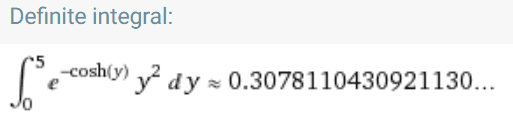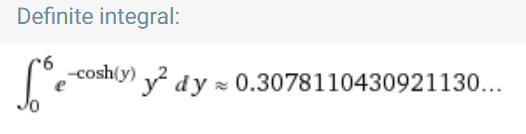with ##k=4##: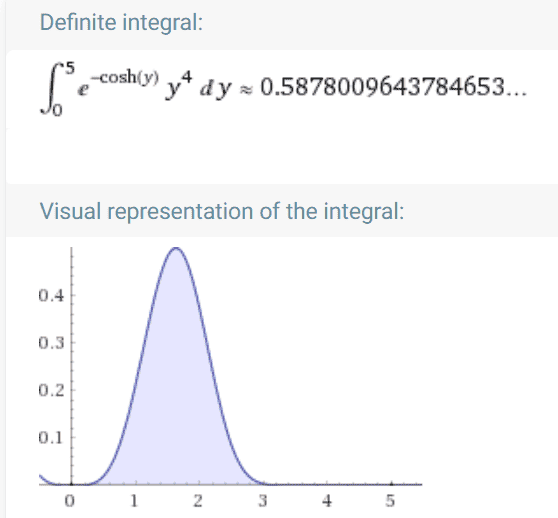(don't trust all digits, though: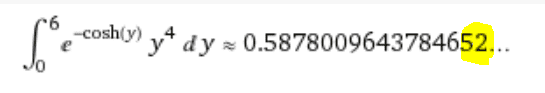and so on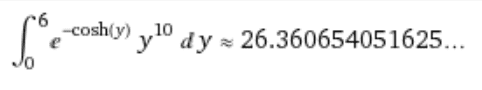But: with larger ##k## things become slippery: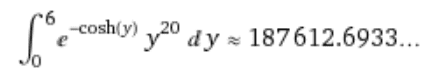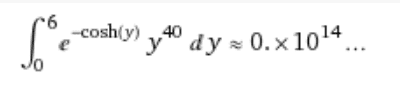So the natural questions are:
• Where does this come from and where is it going ?
• Can you make do with a numerical answer ?
• What precision do you need ?

•Homework Helper
Gold Member
Just so it doesn't become a stumbling point later, one minor correction: ## \cosh{y}=\frac{e^y+e^{-y}}{2} ##, with a 2 in the denominator.

•BvU and Delta2
Homework Helper
2022 Award

I find it hard to believe an analytical answer exists.

Doodled with ##\ u_0=a_0 \ \ \& \ \ u_1 = a_1\ ## so the integral becomes
$$\int y^k\; e^{ -\cosh y}$$ and all the odd ##k## yield zero. What remains is interesting enough:

The general case is $$\int_{-\infty}^\infty (y-C)^k \mathrm{e}^{-\cosh y}\,dy$$ where $$\tanh C = \frac{a_0u_1 - a_1u_0}{a_0u_1 + a_1u_0}$$ It probably has to be done numerically.

Homework Helper
Just so it doesn't become a stumbling point later, one minor correction: ## \cosh{y}=\frac{e^y+e^{-y}}{2} ##, with a 2 in the denominator.
So actually I doodled with ##
\ 2u_0=a_0 \ \ \& \ \ 2u_1 = a_1\
##
The general case is
Can you show how you do that ?

Homework Helper
2022 Award
So actually I doodled with ##
\ 2u_0=a_0 \ \ \& \ \ 2u_1 = a_1\
##
Can you show how you do that ?

That should be $$\int_{-\infty}^\infty (y-C)^k \mathrm{e}^{-D\cosh y}\,dy$$
with $C$ as before and $$D = 2 \sqrt{\frac{u_0u_1}{a_0a_1}}$$

Homework Helper
Still don't see it ...

Homework Helper
2022 Award
Still don't see it ...

Use $e^{\pm y} = \cosh y \pm \sinh y$ and $$D \cosh (y + C) = D \cosh C \cosh y + D \sinh C \sinh y$$ to obtain $$Ae^y + Be^{-y} = (A + B) \cosh y + (A - B) \sinh y = D \cosh(y + C)$$
where $$D \cosh C = A + B),\qquad D\sinh C = A - B.$$ Then substitute $y + C = t$.

But one could go further and use $\int_{-\infty}^\infty f(y)\,dy = \int_0^\infty f(y) + f(-y)\,dy$ to obtain $$\int_0^\infty ((y - C)^k + (-1)^k (y + C)^k)\mathrm{e}^{-D\cosh y}\,dy$$ and expand the polynomials to obtain $$(y - C)^k + (-1)^k (y + C)^k = (-1)^k \sum_{r=0}^k \frac{k!}{(k-r)!r!}C^{k-r} (1 + (-1)^r)y^r$$ from which we see that the terms in odd $r$ vanish and we are left with a linear combination of $\int_0^\infty y^r \mathrm{e}^{-D\cosh y}\,dy$.

Now for large $D$ we can approximate this as $$\mathrm{e}^{-D} \int_0^\infty y^r \mathrm{e}^{-\frac12 Dy^2}\,dy$$ and the substitution $t = \frac12 D y^2$ will turn this into a Gamma function:
$$\int_0^\infty y^r \mathrm{e}^{-\frac12 D y^2}\,dy = \frac{1}{\sqrt{2D}} \left( \frac 2D\right)^{r/2} \int_0^\infty t^{\frac{r-1}2}\mathrm{e}^{-t}\,dt = \frac{1}{\sqrt{2D}} \left( \frac 2D\right)^{r/2}\Gamma\left( \frac{r+1}{2}\right).$$ Thus for large $D$ $$\int_{-\infty}^\infty (y - C)^k \mathrm{e}^{-D\cosh y}\,dy \approx \frac{(-1)^k \mathrm{e}^{-D}}{\sqrt{2D}} \sum_{r=0}^k \frac{k!}{(k-r)!r!} C^{k-r}(1 + (-1)^r) \left(\frac 2D\right)^{r/2} \Gamma\left( \frac{r+1}{2}\right).$$ To leading order only the $r=0$ term is relevant, yielding an estimate of $(-1)^kC^k \mathrm{e}^{-D}\sqrt{2\pi/D}$.

•BvU